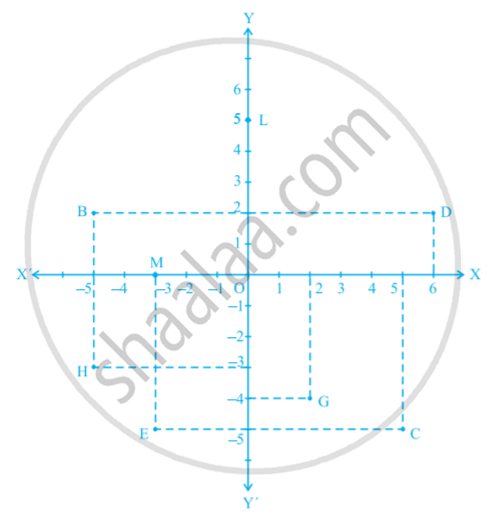# See the given figure, and write the following: The coordinates of the point M. - Mathematics

See the given figure, and write the following:

The coordinates of the point M.#### Solution

The x-coordinate and the y-coordinate of point M are −3 and 0 respectively. Therefore, the coordinates of point M is (−3, 0).

Is there an error in this question or solution?
Chapter 3: Coordinate Geometry - Exercise 3.2 [Page 60]

#### APPEARS IN

NCERT Class 9 Maths
Chapter 3 Coordinate Geometry
Exercise 3.2 | Q 2. (viii) | Page 60

Share